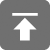# 工学1号馆

home

## Project Euler 33–Digit canceling fractions

Wu Yudong    August 20, 2018     欧拉计划   489

The fraction 49/98 is a curious fraction, as an inexperienced mathematician in attempting to simplify it may incorrectly believe that 49/98 = 4/8, which is correct, is obtained by cancelling the 9s.

We shall consider fractions like, 30/50 = 3/5, to be trivial examples.

There are exactly four non-trivial examples of this type of fraction, less than one in value, and containing two digits in the numerator and denominator.

If the product of these four fractions is given in its lowest common terms, find the value of the denominator.

49/98是一个有趣的分数，因为缺乏经验的数学家可能在约简时错误地认为，等式49/98 = 4/8之所以成立，是因为在分数线上下同时抹除了9的缘故。

//（Problem 33）Digit canceling fractions
// Completed on Thu, 25 Jul 2013, 17:47
// Language: C
//
// 版权所有（C）wu yudong
// 博客地址：http://www.wuyudong.com

#include<stdio.h>
void swap(int *a, int *b)
{
int t;
t = *a;
*a = *b;
*b = t;
}

int gcd(int a, int b)
{
int r;
if (a < b)
swap(&a, &b);
if (!b)
return a;
while ((r = a % b) != 0) {
a = b;
b = r;
}
return b;
}

void find()
{
int i;
int M, N;
M = N = 1;
for (i = 12; i < 50; i++) {
for (int j = i + 1; j < 100; j++) {
int t = gcd(i, j);
if (t == 1 || i / t > 10 || j / t > 10 || i % 10 != j / 10)
continue;
else {
int a = i / 10, b = j % 10;
if (a / gcd(a, b) == i / t && b / gcd(a, b) == j / t) {
M *= i / t;
N *= j / t;
}
}
}
}
printf("%d\n", N / gcd(M, N));
}

int main()
{
find();
return 0;
}